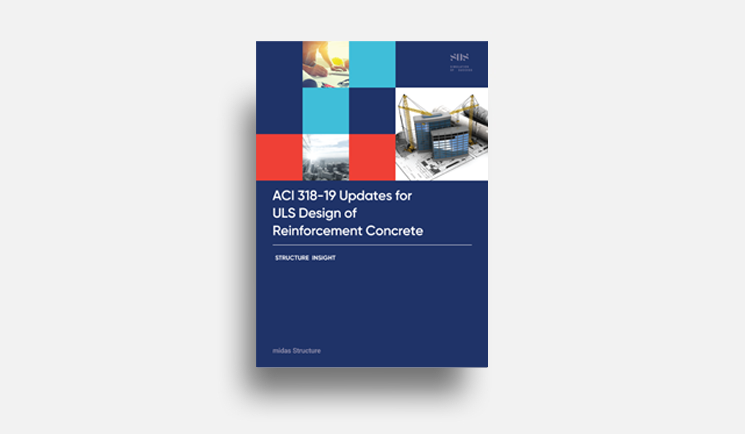# midas Structure

BLOG PROJECT TUTORIAL

# What is it? - Deflection Limitation

The beam deflection is one of the checks that should be performed for serviceability limit state design. Deflection is the displacement within a structural member under the influence of loads, ignoring the displacements of the rest of the structure.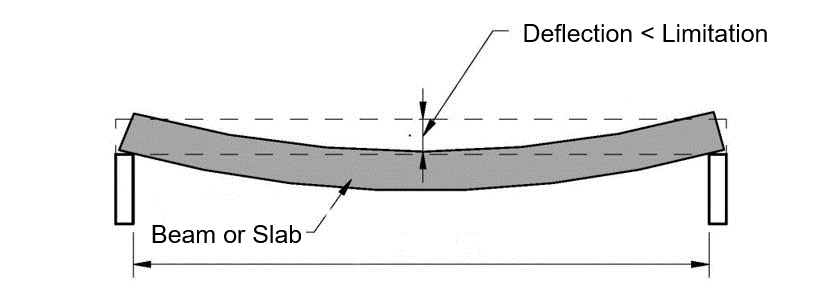Figure 1. Deflection Limitation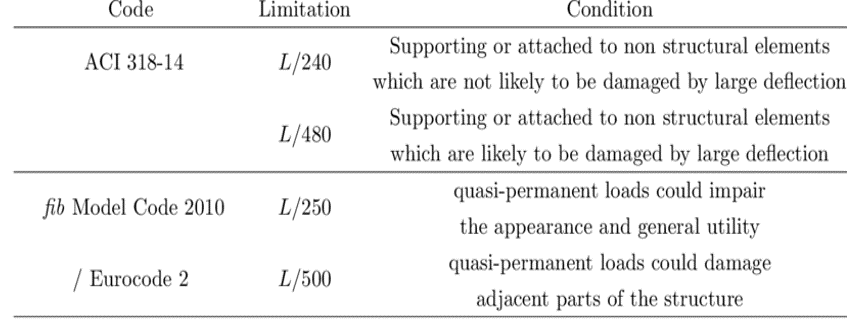Figure 2. Suggested Deflection Limits for Structural Elements

# Calculation of Stiffness Center

## How to calculate it? (Comparison of Analysis Result & Design Result)

SW does not simply use analysis results because the deflection in the analysis result is a value including the deformation of adjacent members.

## Design result of the cantilever beam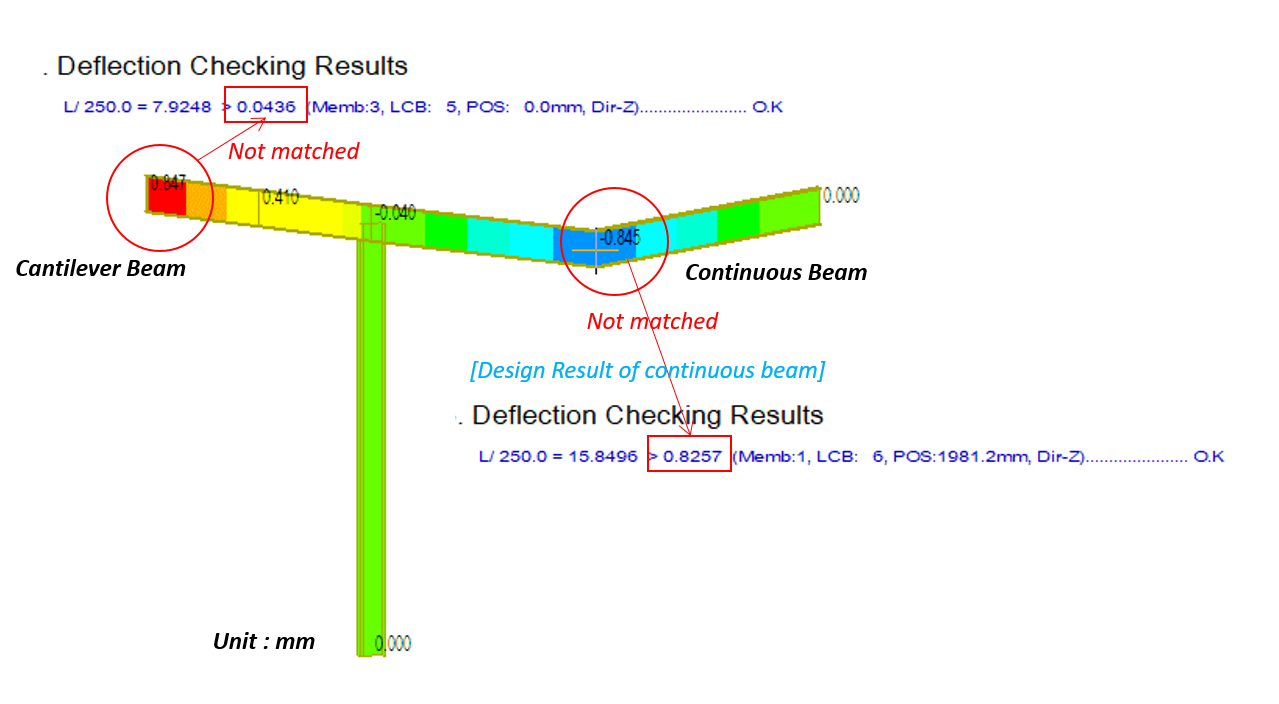Figure 3. Deflection Checking Results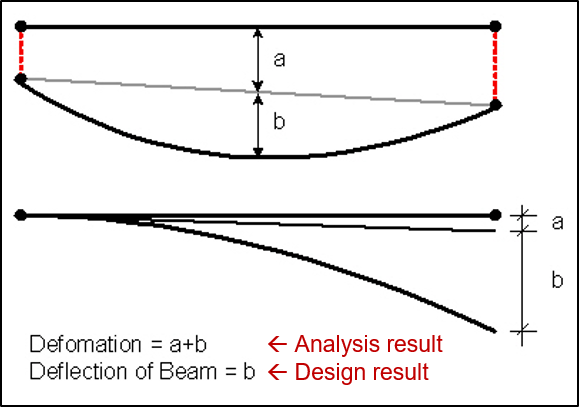Figure 4. Analysis and Design Results

## How to calculate it by manual? (Example - Cantilever)

Calculation by manual gives an approximate value, so manual value and Gen result value cannot be matched.

Please refer only to the calculating concept and way.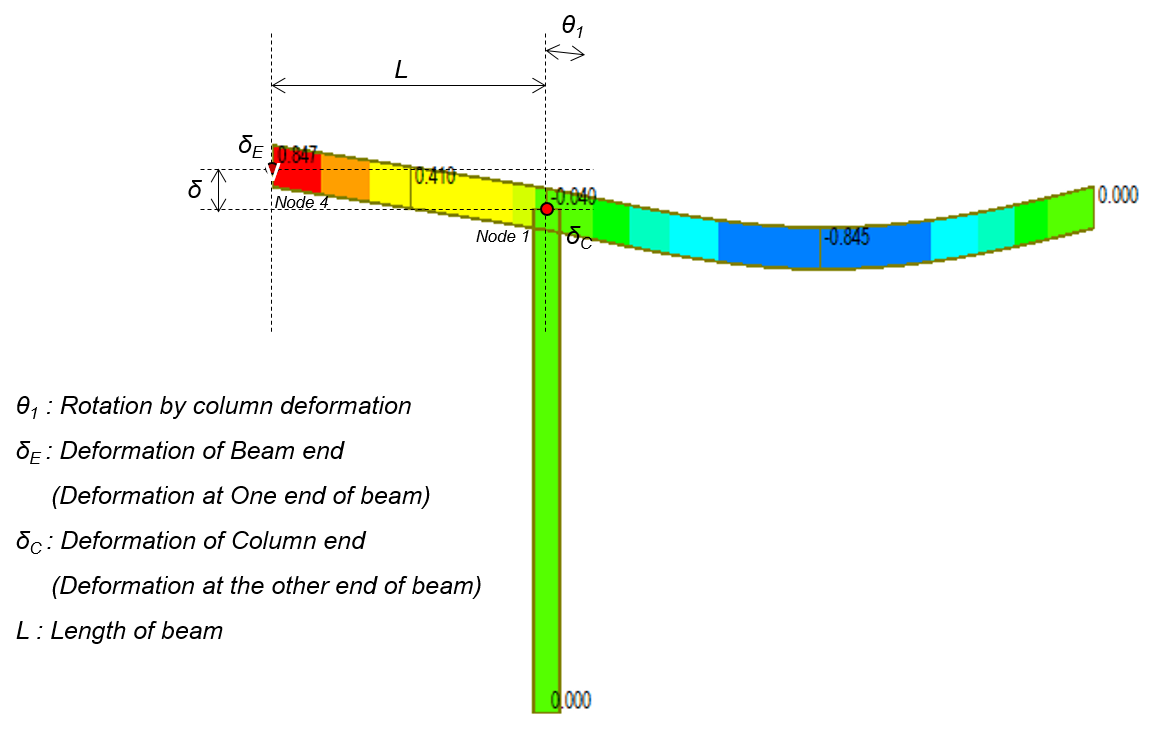Figure 5. Cantilever Example Model

## How to calculate the deformation of the cantilever by manual (unit : mm) - Example

δ = (δE –δC ) – θ1 *L (add this to remove the effect by column deformation)

= (0.846566 – (-0.039507)) – 0.000473* 1981.2

= -0.0510 mm

## Deflection checking results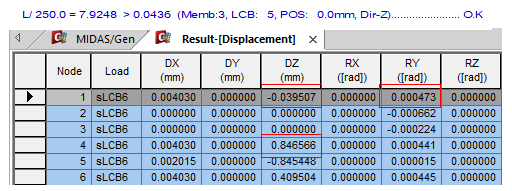Figure 6. Deflection Checking Results - 1

## How to calculate it through Gen? (Example - Cantilever)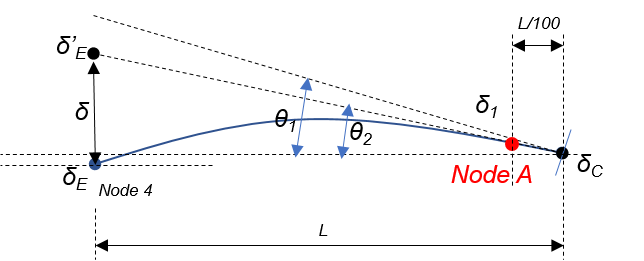Figure 7. Example Cantilever Model with Node A

θ1 : Rotation by column deformation

θ2 : The angle of the line that links the deformed points of the

beam-column joint and of first node when L is divided into

100 equal parts.

δE : Deformation of Beam end (Deformation at One end of beam)

δC : Deformation of Column end (Deformation at the other end of

beam)

Node A : the first internal node when L is divided into 100 equal

parts

L : Length of beam

## How to calculate deformation of the cantilever in midas Gen (unit : mm)

Gen uses θ2  calculated internally. θ2 means the angle of the line connecting the nodes of the column and the deformed node closest to the column when the beam is divided into 100 equal parts and

θ2 = (Dz at node A = Dz at Node 1 ) / (L/100)

=  (-0.031082 – (-0.039507)) / (1981.2/100)

= 0.000425

δ = (δE δC ) –θ2 *L

= (0.846566 – (-0.039507)) – 0.000425* 1981.2

= - 0.0436 mm

## Deflection checking results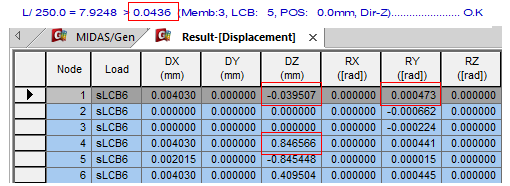Figure 6. Deflection Checking Results - 1

Dz at Node A = -0.031082

Since this is an internal value, it is not output separately.

## How to calculate it? (Example -Continous Beam)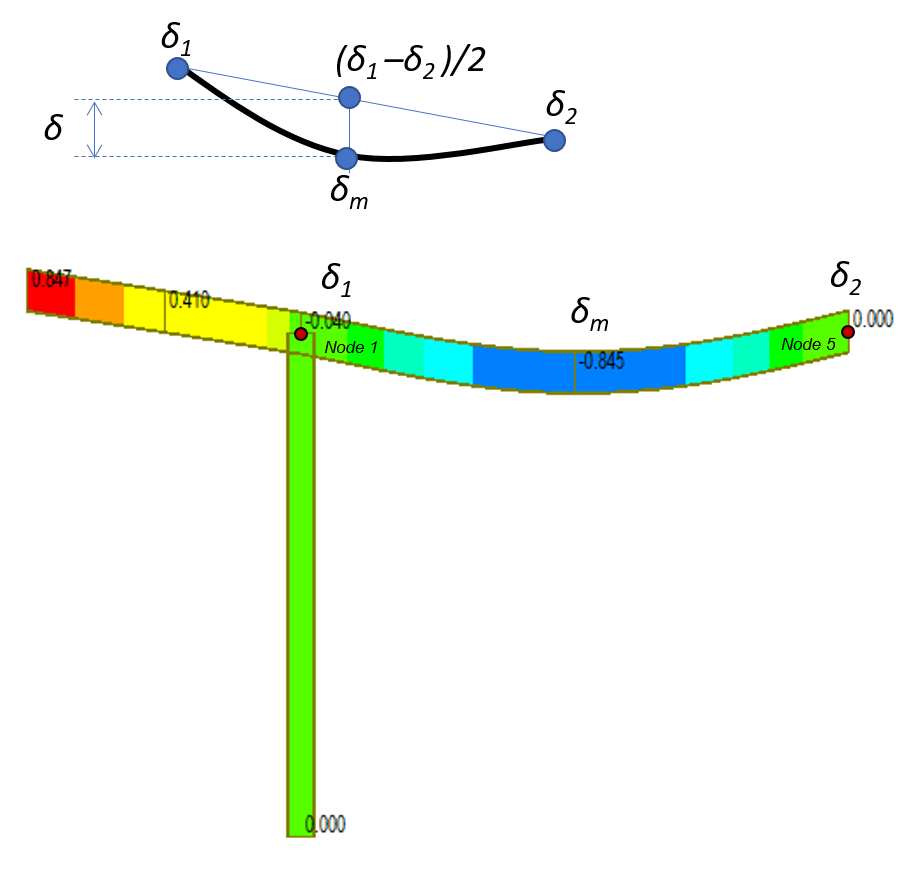Figure 8. Continuous Beam Example Model

δ1 : Deformation at One end of beam

δ2 : Deformation at the other end of beam

δm : Deformation at middle point of beam

## How to calculate the deformation of the cantilever by manual (unit : mm)

δ = δm – (δ1 δ2 )/2

= 0.845448 – (0.039507 – 0.000) / 2

= 0.8257 mm

## Deflection checking results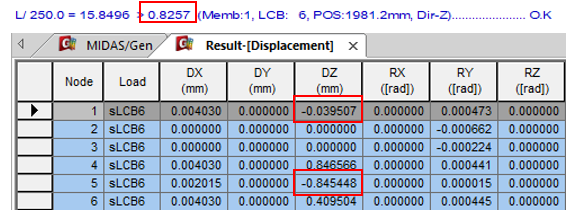Figure 9. Deflection Checking Results -2

Author InformationYeong-il Seo | Principal Structural Engineer

Young-il has over 13+ years of experience in building design, especially high-rise buildings with column reduction analysis, plant structures, pushover analysis, health monitoring, and vibration control projects. Since 2016, he is planning and providing technical supports for midas building products such as midas Gen, nGen, and Design+.